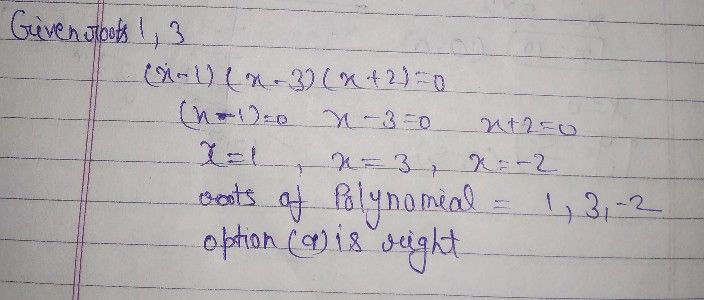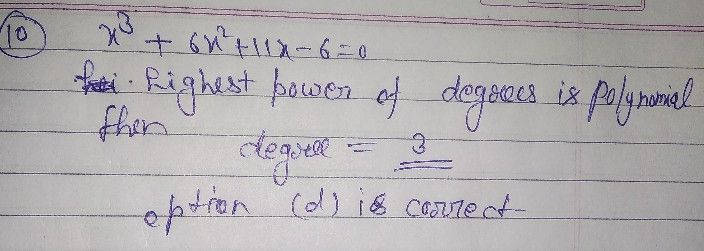Symbol
Problem$9.$ If $1,3$ are the roots of the polynomial equation $\left(x-1\right)\left(x-3\right)\left(x+2\right)$ $=0$ What is the other roots ? a. $-2$ b. $2$ C. d. $-3$ $-10$ What is the degree of the polynomial equation $x^{3}+6x^{2}+11x$ $-6=0$ a. $2$ b. $4$ C. d. $3$ $11$ Which of the following is NOT a factor of $P\left(x\right)=x^{3}-x^{2}-10x-87$ a. $x-1$ b. $x+1$ C. $x-4$ d. $x+2$
10th-13th grade
Other
Search count: 106
SolutionQanda teacher - Deepakplease give me review by 5 ?
one time one question allow
this is qanda rule
please give me review by 5 ?